Function Repository Resource:

# KirchhoffTensor

Compute the Kirchhoff tensor (Laplacian) of an arbitrary hypergraph

Contributed by: Jonathan Gorard
 ResourceFunction["KirchhoffTensor"][h] gives the Kirchhoff tensor of the (ordered or orderless) hypergraph h.

## Details and Options

A Kirchhoff tensor (hypergraph Laplacian) is a generalization of the concept of a Kirchhoff matrix (graph Laplacian) from graphs to hypergraphs, in which hyperedges may be of arbitrary arity.
The rank of the Kirchhoff tensor is equal to the arity of the hyperedges of the hypergraph.
The Kirchhoff tensor for a hypergraph will have dimensions n×n××n, where n is the number of vertices.
ResourceFunction["KirchhoffTensor"] returns a SparseArray object, which can be converted into ordinary nested lists using Normal.
The diagonal entries aii…i represent the degrees for each vertex vi.
An off-diagonal entry aij…k is equal to -1 if vertices vi,vj,,vk are adjacent (or, in the case of multihypergraphs, -aij…k is equal to the number of hyperedges of the form {vi,vj,,vk}).
ResourceFunction["KirchhoffTensor"] has the following options:
 "OrderedHyperedges" False whether to treat hyperedges as being ordered (directed) "OrderedHyperedgeDegree" "InOutDegree" which notion of vertex degree to use for ordered (directed) hypergraphs
The following settings for "OrderedHyperedgeDegree" can be used in ResourceFunction["KirchhoffTensor"]:
 "InOutDegree" treat the degree of a vertex in an ordered (directed) hypergraph as the sum of its in- and out-degrees "InDegree" treat the degree of a vertex in an ordered (directed) hypergraph as its in-degree "OutDegree" treat the degree of a vertex in an ordered (directed) hypergraph as its out-degree
When all hyperedges are of arity 2, the output of ResourceFunction["KirchhoffTensor"] is identical to that of KirchhoffMatrix.

## Examples

### Basic Examples (3)

The Kirchhoff tensor of an orderless hypergraph, with hyperedges of arity 3:

 In:=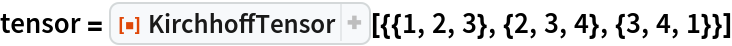Out=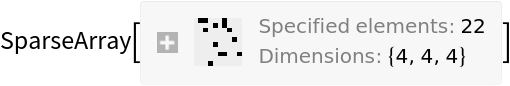In:=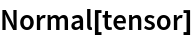Out=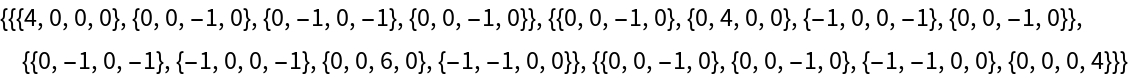The Kirchhoff tensor of an ordered hypergraph, with hyperedges of arity 3:

 In:=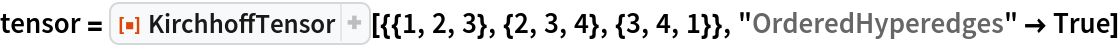Out=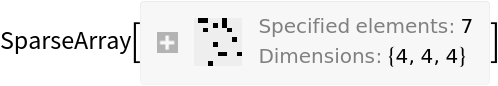In:=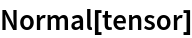Out=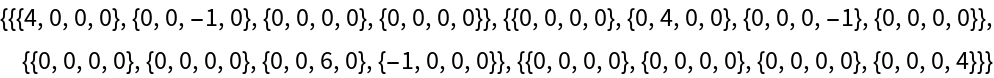Use only vertex in-degrees along the main diagonal:

 In:=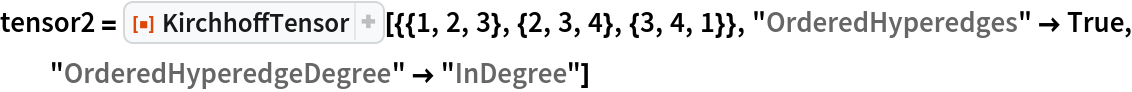Out=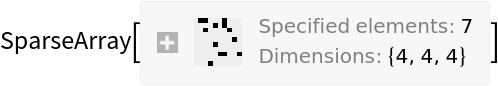In:=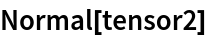Out=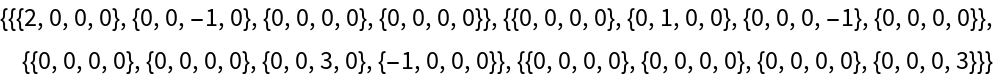The Kirchhoff tensor of an orderless hypergraph, with hyperedges of arity 5:

 In:=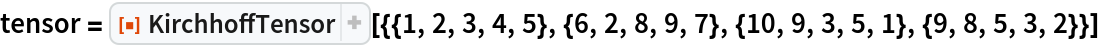Out=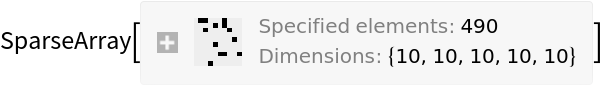### Scope (6)

KirchhoffTensor supports multihypergraphs, in which case the off-diagonal tensor entries represent hyperedge multiplicities:

 In:=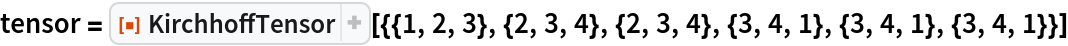Out=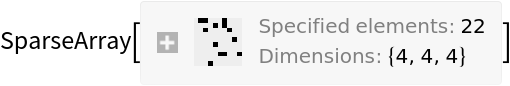In:=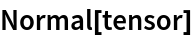Out=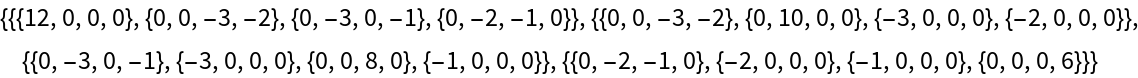When the arity of hyperedges is equal to 2, the output of KirchhoffTensor is identical to the output of KirchhoffMatrix:

 In:=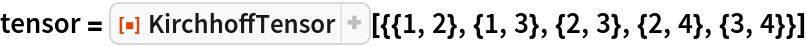Out=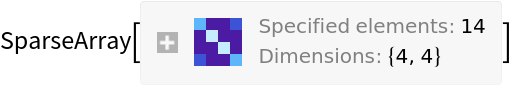In:=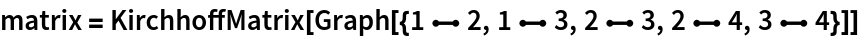Out=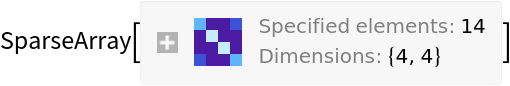In:=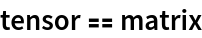Out=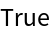The Kirchhoff tensor of an orderless hypergraph is always symmetric across all indices:

 In:=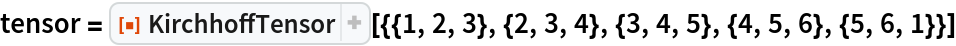Out=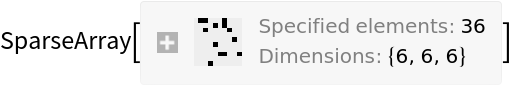In:=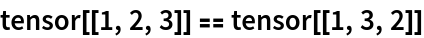Out=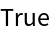The Kirchhoff tensor of an ordered hypergraph is not necessarily symmetric across all indices:

 In:=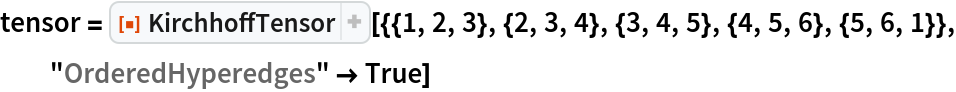Out=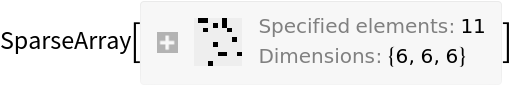In:=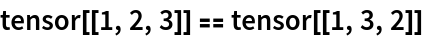Out=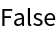KirchhoffTensor automatically removes self-loops from a hypergraph:

 In:=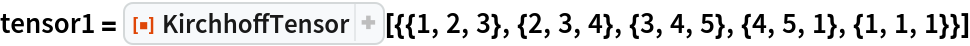Out=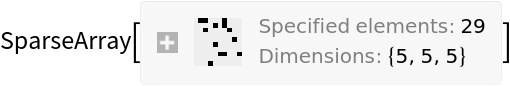In:=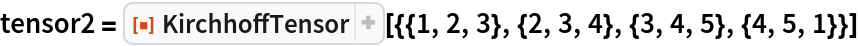Out=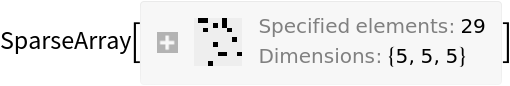In:=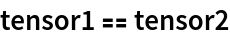Out=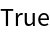Hyperedges can be of arbitrary arity:

 In:=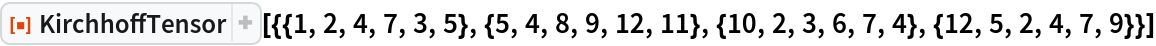Out=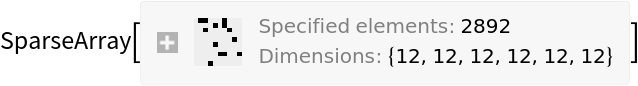### Options (5)

#### Ordered/orderless hyperedges (2)

By default, all hyperedges are treated as orderless (i.e. undirected):

 In:=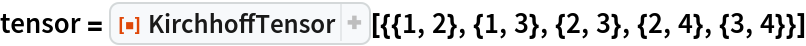Out=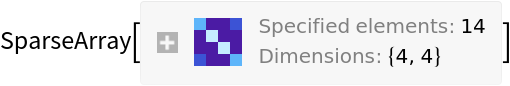In:=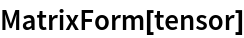Out=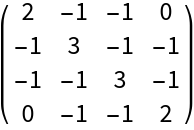Use "OrderedHyperedges"True to treat hyperedges as ordered (i.e. directed):

 In:=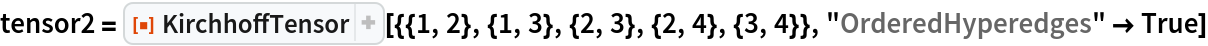Out=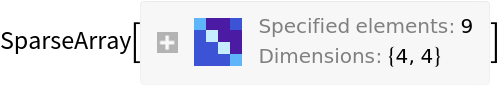In:=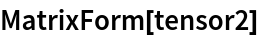Out=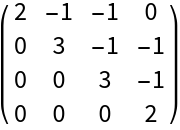#### Ordered hyperedge degrees (3)

By default, the vertex degrees along the main diagonal for an ordered (i.e. directed) hypergraph are given by the sum of vertex in- and out-degrees:

 In:=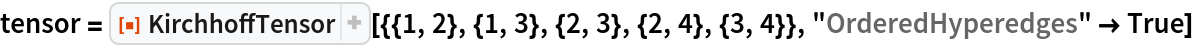Out=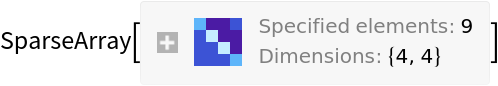In:=Out=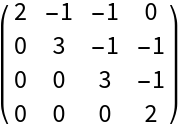Use "OrderedHyperedgeDegree""InDegree" to use the vertex in-degree instead:

 In:=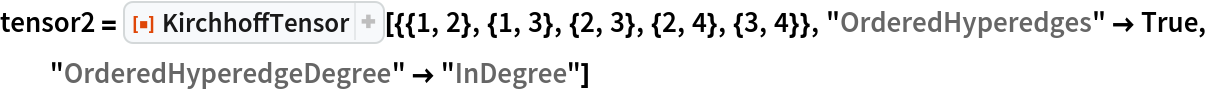Out=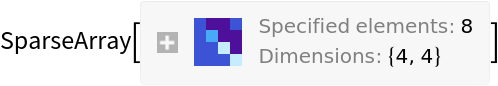In:=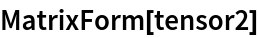Out=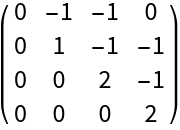Use "OrderedHyperedgeDegree""OutDegree" to use the vertex out-degree instead:

 In:=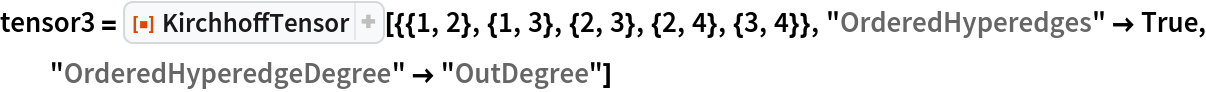Out=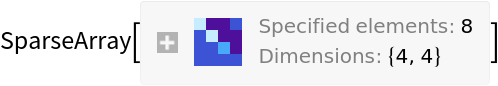In:=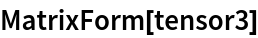Out=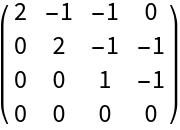Jonathan Gorard

## Version History

• 1.0.0 – 17 March 2021